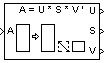# Singular Value Decomposition

Factor matrix using singular value decomposition

## Library

Math Functions / Matrices and Linear Algebra / Matrix Factorizations

`dspfactors`

•## Description

The Singular Value Decomposition block factors the M-by-N input matrix A such that

`$A=U\cdot diag\left(S\right)\cdot {V}^{*}$`

where

• U is an M-by-P matrix

• V is an N-by-P matrix

• S is a length-P vector

• P is defined as min(M,N)

When

• M = N, U and V are both M-by-M unitary matrices

• M > N, V is an N-by-N unitary matrix, and U is an M-by-N matrix whose columns are the first N columns of a unitary matrix

• N > M, U is an M-by-M unitary matrix, and V is an N-by-M matrix whose columns are the first M columns of a unitary matrix

In all cases, S is an unoriented vector of positive singular values having length P.

Length-N row inputs are treated as length-N columns.

Note that the first (maximum) element of output S is equal to the 2-norm of the matrix A.

## Parameters

Show singular vector ports

Select to enable the `U` and `V` output ports.

Show error status port

Select to enable the E output port, which reports a failure to converge. The possible values you can receive on the port are:

• `0` — The singular value decomposition calculation converges.

• `1` — The singular value decomposition calculation does not converge.

If the singular value decomposition calculation fails to converge, the output at ports U, S, and V are undefined matrices of the correct size.

Simulate using

Type of simulation to run. You can set this parameter to:

• `Interpreted execution` (default)

Simulate model using the MATLAB®  interpreter. This option shortens startup time.

• `Code generation`

Simulate model using generated C code. The first time you run a simulation, Simulink® generates C code for the block. The C code is reused for subsequent simulations, as long as the model does not change. This option requires additional startup time.

## References

Golub, G. H., and C. F. Van Loan. Matrix Computations. 3rd ed. Baltimore, MD: Johns Hopkins University Press, 1996.

## Supported Data Types

PortSupported Data Types

A

• Double-precision floating point

• Single-precision floating point

U

• Double-precision floating point

• Single-precision floating point

S

• Double-precision floating point

• Single-precision floating point

V

• Double-precision floating point

• Single-precision floating point

E

• Boolean

 Autocorrelation LPC DSP System Toolbox Cholesky Factorization DSP System Toolbox LDL Factorization DSP System Toolbox LU Inverse DSP System Toolbox Pseudoinverse DSP System Toolbox QR Factorization DSP System Toolbox SVD Solver DSP System Toolbox `svd` MATLAB